# Texas Go Math Grade 4 Lesson 12.1 Answer Key Number Patterns

Refer to our Texas Go Math Grade 4 Answer Key Pdf to score good marks in the exams. Test yourself by practicing the problems from Texas Go Math Grade 4 Lesson 12.1 Answer Key Number Patterns.

## Texas Go Math Grade 4 Lesson 12.1 Answer Key Number Patterns

Essential Question

How can you make and describe patterns?
A pattern is a sequence of numbers or objects, a repeated patterns with a set of rule.
we can make and describe patterns of numbers by finding and using rule to follow.
we look at the given information and rule to create a pattern.
Explanation:
For example;
Rule Add 5 first term t =3
r = t + 5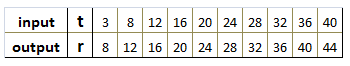Unlock the Problem

Daryl is making a pattern for a quilt. The pattern shows 40 squares. Every fourth square is blue. How many blue squares are in the pattern?
Underline what you are asked to find.
Circle what you need to use.
A pattern is an ordered set of numbers or objects. Each number or object in the pattern is called a term.
10 blue patternsExplanation:
Rule add 4 to first term
Input a = 4,8,12,16,20,24,28,32,36
Output b = a + 4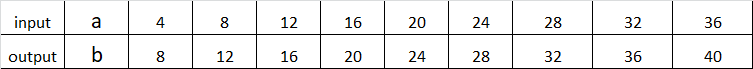Activity Find a pattern.

Materials

• color pencils

Shade the squares that are blue.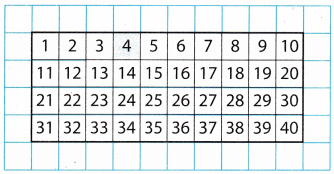Which squares are blue? ______________
So, there are __________ blue squares in the pattern.
Which squares are blue 4 , 8 , 12 , 16 , 20 , 24, 28, 32, 36, 40
So, there are 10 blue squares in the pattern.
Explanation:
Rule Add 4 to first termMath Talk

Mathematical Processes
Describe another number pattern in Daryl’s quilt.
Rule add 5 to first term
Input a = 5,10,15, 20,25, 30,35, 40, 45, 50.
Output b = a + 5Explanation:
Daryl is making a pattern for a quilt.
The pattern shows 50 squares.
Every fifth square is blue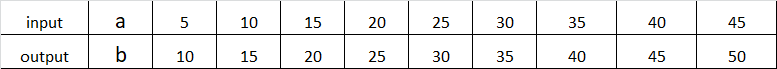Question 1.
What patterns do you see in the arrangement of the blue squares?
The pattern shows 50 squares. Every fifth square is blueExplanation:
Rule: Output +5
a = 5, 10, 15, 20, 25, 30, 35, 40, 45, 50
b = a + 5
Question 2.
What patterns do you see in the numbers of the blue squares?
Pattern – I Add 4 to the first term.
Pattern – II Add 5 to the first term.

Example Find and describe a pattern.

The rule for the pattern is add 5. The first term in the pattern is 5.

A. Use the rule to write the numbers in the pattern.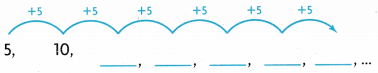Rule: Add 5 to the first term.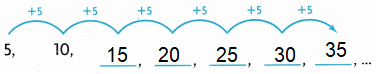Explanation:
Rule: Add 5 to the first term.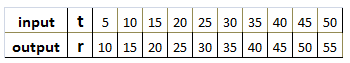5 + 5 = 10
10 + 5 = 15
15 + 5 = 20
20 + 5 = 25
25 + 5 = 30
30 + 5 = 35

B. Describe other patterns in the numbers.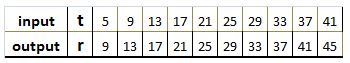Explanation:
Rule: Add 4 to first term t= 5
r = t + 4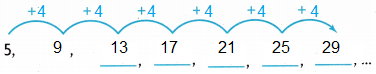What do you notice about the digits in the ones place?
5 in ones place is increasing  by 4,
first term is t + 4 = r
r = t + 4

Describe the pattern using the words odd and even.
For odd pattern
t= 1 and r = t+2
for even patterns
t=2, and r = t+2
Explanation: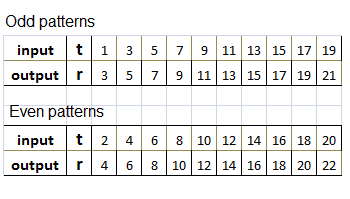Share and Show

Use the rule to write the numbers in the pattern.

Question 1.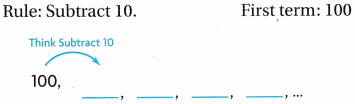Explanation:
Rule subtract 10 from the first term to continue the pattern
100 – 10 = 90
90 – 10 = 80
80 – 10 = 70
70 – 10 = 60

Math Talk

Mathematical Processes
Explain how the first term in a pattern helps you find the next term.
A pattern is a sequence of numbers of repeated patterns with a set of rule.
The first number is the starting point and rule is to find the next number
For example;
Rule Add 4 first term t =5Use the rule to write the numbers in the pattern.
Describe another pattern in the numbers.

Question 2.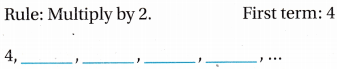Explanation:
Rule: Multiply the first term with 2
4 x 2 = 8
8 x 2 = 16
16 x 2 = 32
32 x 2 = 64

Question 3.Explanation:
Rule: skip counting by 6 from the first term,
we skip 6 numbers for the next term.

Question 4.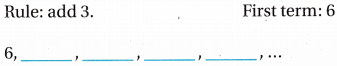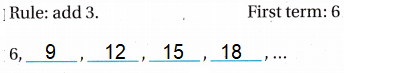Explanation:
Rule: Add 3 to the first term
6 + 3 = 9
9 + 3 = 12
12 + 3 = 15
15 + 3 = 18

Problem Solving

Question 5.
Analyze The odd and even-numbered hotel rooms are on different sides of the hall. Room 231 is between which two rooms?
Room 231 is between 229 and 233
Explanation:
Arrange the numbers left and right of the given number 231as below,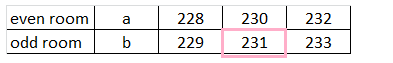b = a + 1

Question 6.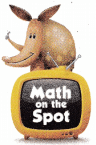H.O.T. Multi-Step John is saving for his trip to see the Alamo. He started with $24 in his savings account. Every week he earns$15 for baby-sitting. Out of that, he spends $8 and saves the rest. John uses the rule add 7 to find out how much money he has at the end of each week. What are the first 8 numbers in the pattern? Answer: b = a + 7first term is a = 24 second term b = a + 7 Explanation: John started with$24 in his savings account.
Every week he earns $15 for baby-sitting. Out of that, he spends$8 and saves the rest.
John uses the rule add 7 with the first 8 numbers in the pattern.
Question 7.
H.O.T. Multi-Step Pose a Problem An activity at the Math Fair shows two charts.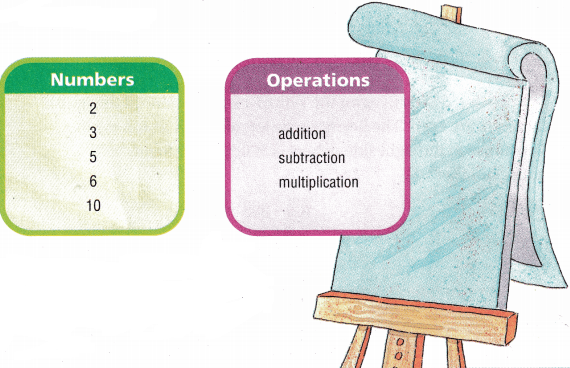Use at least two of the numbers and an operation from the charts to write a pattern problem. Include the first five terms of your pattern in the solution to your problem.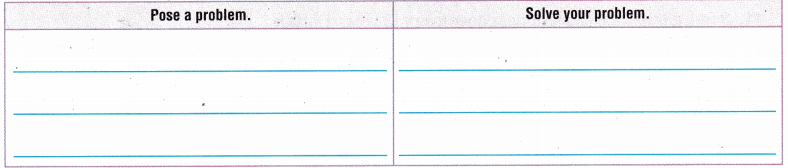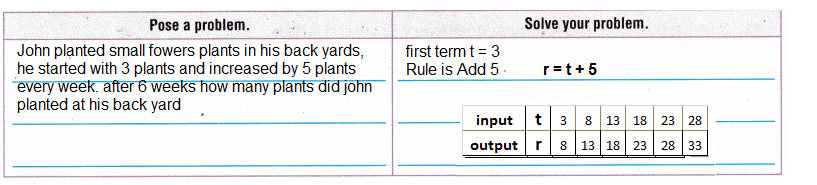Explanation:
Rule: Add 5 to the first term 3
Input t = 3, 8, 13, 18, 23, 28
Output r = t + 3

Describe other patterns in the terms you wrote.
first term is t = 3
Rule taken is Add 5 , r = t + 5
Operation taken from chart is Addition
Explanation:
A pattern is a sequence of numbers or objects, a repeated patterns with a set of rule.
we can make and describe patterns of numbers by finding and using rule to follow.
we look at the given information and rule to create a pattern

Question 8.
Which pattern follows the rule add 3?
(A) 60, 63, 60, 63, ……
(B) 3, 1, 4, 2,…
(C) 60, 63, 62, 65,…
(D) 60, 63, 66, 69,…
Option(D)
Explanation:
b = a + 3
b = 60 + 3 = 63
b = 63 + 3 = 66
b = 66 + 3 = 69

Question 9.
The rule for a pattern is add 5. The first term in the pattern is 6. Which shows the numbers in the pattern?
(A) 6, 11, 9, 14, 12, …….
(B) 6, 11, 16, 21, 26,……
(C) 5, 10, 8, 13, 11,……..
(D) 5, 2, 10, 8, 15,…..
Option(B)
Explanation:
Rule: Add 5 to the first term 6
t = 6,
r = t + 5
r = 6 + 5 = 11
r = 11 + 5 = 16
r = 16 + 5 = 21
r = 21 + 5 = 26

Question 10.
Multi-Step Sandy lives on the side of a Street where all the house numbers are even. The house to the left of hers is number 356. The house to the right side of hers is 360. What is Sandy’s house number?
(A) 354
(B) 358
(C) 362
(D) 357
Option (B)
Explanation:
Rule: Add 2 to the given term 356
r = t + 2
t = 356
r = 356 + 2 = 358
r = 358 + 2 = 360

TEXAS Test Prep

Question 11.
Some groups of cicadas appear once every 13 years. Darla is 5 when she hears her first group of cicadas. Which pattern shows her ages the next 4 times that a group of cicadas appears?
(A) 13, 26, 39, 52
(B) 13, 18, 23, 28
(C) 18,31,44,57
(D) 18,23,28,33
Option (C)
Explanation:
Some groups of cicadas appear once every 13 years.
Darla is 5 when she hears her first group of cicadas.
The below pattern shows her ages the next 4 times that a group of cicadas appears
a = 5
b = a + 13
b = 5 + 13 = 18
b = 18 + 13 = 31
b = 31 + 13 = 44
b = 44 + 13 = 57

### Texas Go Math Grade 4 Lesson 12.1 Homework and Practice Answer Key

Use the rule to write the numbers in the pattern.

Question 1.Explanation:
t = 50
r = t – 5
r = 50 -5 = 45
r = 45 – 5 = 40
r = 40 – 5 = 35
r = 35 – 5 = 30

Question 2.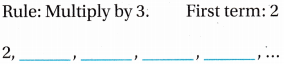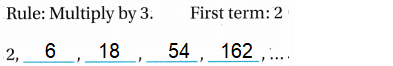Explanation:
t = 2
r = t x 3
r = 2 x 3 = 6
r = 6 x 3 = 18
r = 18 x 3 = 54
r = 54 x 3 = 162

Use the rule to write the numbers in the pattern.
Describe another pattern in the numbers.

Question 3.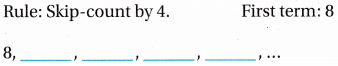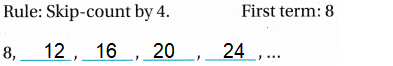Explanation:
t = 8
r = t + 4
r = 8+4 = 12
r = 12+4 = 16
r = 16+4 = 20
r = 20+4 = 24

Question 4.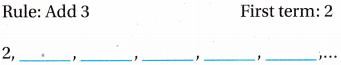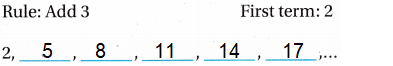Explanation:
t = 2
r = t + 3
r = 2 + 3 = 5
r = 5 + 3 = 8
r = 8 + 3 = 11
r = 11 + 3 = 14
r = 14 + 3 = 17

Problem Solving

Question 5.
Kate’s hair is currently 10 inches long. She read that her hair grows about 6 inches per year. Kate uses the rule add 6 10 find out how long her hair would be in 4 years if she does not cut it. What are the first 5 numbers in the pattern?
Rule: Add 6 to the first term 10Explanation:
Kate’s hair is currently 10 inches long.
The first 5 numbers in the pattern
t = 10
r = t + 6
r = 10 + 6 = 16
r = 16 + 6 = 22
r = 22 + 6 = 28
r = 28 + 6 = 34
r = 34 + 6 = 40

Question 6.
After not raining for weeks, John measured 2 inches of rainfall in his rain gauge on Monday. On Tuesday, 2 more inches fell. John uses the rule add 2 to find out how many inches of rainfall he will measure if the pattern continues. What are the first 4 numbers in the pattern?Explanation:
Rule: Add 2 to the first term 2
t = 2
r = t + 2
Monday 2 inches rain fall
Tuesday 2 more inches rain fell
2 + 2 = 4
total 10 inches rain fall is measured , as shown in the above table.

Lesson Check

Question 7.
The rule for a pattern is add 4. The first 8 term in the pattern is 4. Which shows the numbers in the pattern?
(A) 4, 8, 12, 16, 20
(B) 4, 1, 5, 2, 6
(C) 5, 20, 80, 160, 640
(D) 5, 9, 13, 17, 21
Option(A)
Explanation:
Rule: Add 4 to the first term 8
t = 4
r = t + 4
r = 4 + 4 = 8
r = 8 + 4 = 12
r = 12 + 4 = 16
r = 16 + 4 = 20

Question 8.
Cassie’s family has a reunion every 3 years. Cassie was 4 at her first family reunion. Which pattern shows Cassie’s age for the next 5 family reunions?
(A) 7, 10, 13, 16, 19
(B) 9, 14, 19, 24,29
(C) 8,12,16,20,24
(D) 9, 12, 15, 18,21
Option(A)
Explanation:
Rule: Add 3 to the first term 4
t = 3
r = t + 4
r = 4 + 3 = 7
r = 7 + 3 = 10
r = 10 + 3 = 13
r = 13 + 3 = 16
r = 16 + 3 = 19

Question 9.
Which pattern shows the rule multiply by 4?
(A) 4,8,12,16…
(B) 1,4,16,64…
(C) 4,12,36,108…
(D) 10,14,18,22…
Option (B)
Explanation:
Rule: Multiply by 4
t = 1
r = t x 4
r = 1 x 4 = 4
r = 4 x 4 = 16
r = 16 x 4 = 64

Question 10.
Rahul has saved $86 from his summer job. When school begins, he plans to spend$5 each week. Which pattern shows how much money Rahul will have after 4 weeks?
(A) $82,$78, $74,$70
(B) $81,$77, $72, S68 (C)$82, $77,$72, $67 (D)$81, $76,$71, $66 Answer: Option(D) Explanation: Rahul has saved$86 from his summer job.
When school begins, he plans to spend $5 each week. The pattern shows total money Rahul have after 4 weeks Rule: Subtract 5 from the first term 86 t = 86 b = a – 5 b = 86 – 5 = 81 b = 81 – 5 = 76 b = 76 – 5 = 71 b = 71 – 5 = 66 Question 11. Multi-Step Paul earns$7 each week walking his neighbor’s dog. Each week, he subtracts $3 from his earnings to spend and saves the rest. Which pattern shows the amount that Paul will have in his savings after 6 weeks? (A)$4, $8,$12, $16,$20, $24 (B)$7, $14,$21, $28,$35, $42 (C)$4, $7,$11, $18,$22, $26 (D)$7,$10,$13,$16,$19$22 Answer: Option (A) Explanation:Paul earns$7 each week walking his neighbor’s dog.
Each week, he subtracts \$3 from his earnings to spend and saves the rest.
The pattern shows the amount that Paul have in his savings after 6 weeks
Rule: Subtract 3 from the first term 7
t = 7
r = t – 3
r = 7 – 3 = 4
r = t – 3 = 4

Question 12.
Multi-Step Marcie likes to collect stickers, but she also likes to give them away. Currently, Marcie has 87 stickers in her collection. If Marcie collects 5 new stickers each week and gives away 3 stickers each week, which of the following patterns shows how many stickers Marcie will have in her collection after 5 weeks?
(A) 87, 84, 81, 78, 75, 72
(B) 87, 89, 91, 93, 95, 97
(C) 87, 92, 97, 102, 107, 112
(D) 87, 95, 103, 111, 119, 127
Option (B)
Explanation:
Marcie has 87 stickers in her collection.
If Marcie collects 5 new stickers each week and gives away 3 stickers each week,
The following patterns shows Marcie stickers collection after 5 weeks
Rule: Add 5 and subtract 3 to the first term 87
t = 87
r = t + 5 – 3
r = 87 + 2 = 89
r = 89 + 2 = 91
r = 91 + 2 = 93
r = 93 + 2 = 95
r = 95 + 2 = 97

Scroll to Top